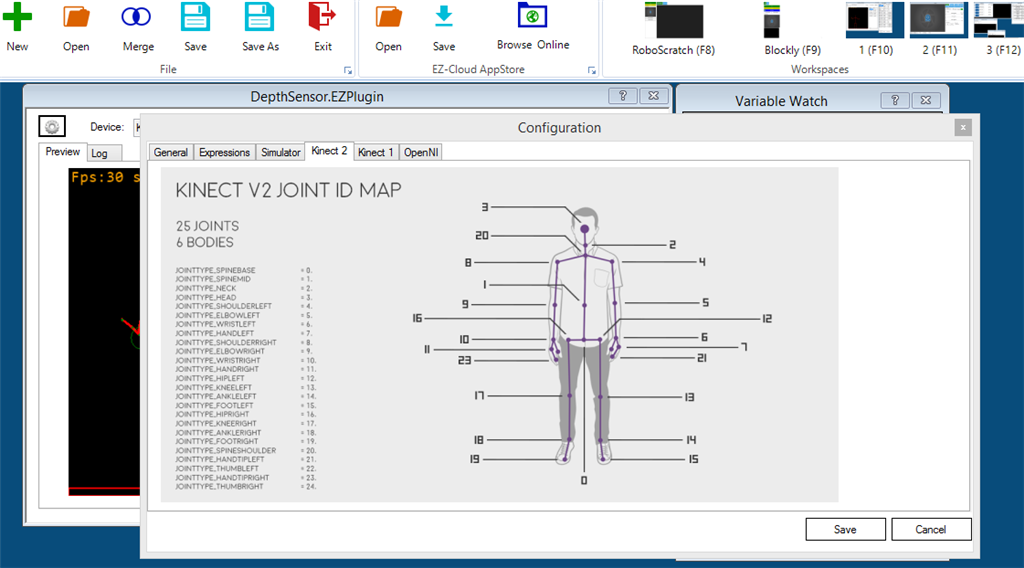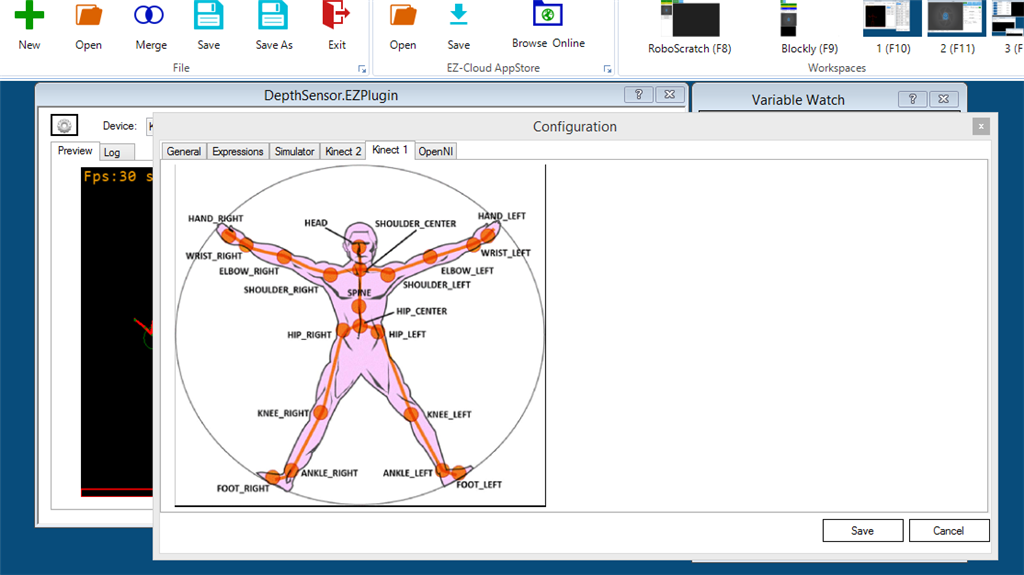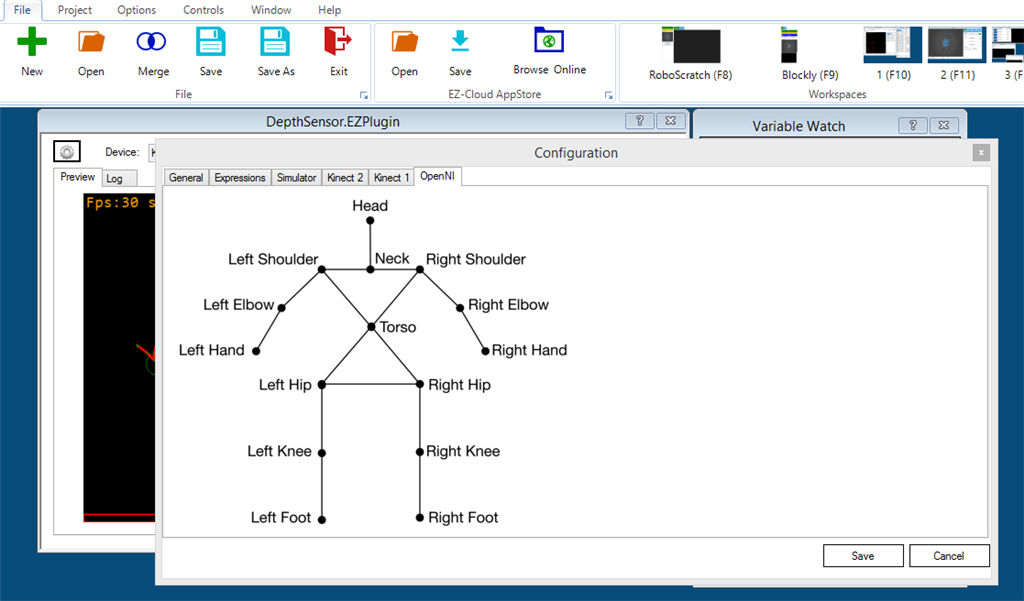Behavior Control
This control is developed by:
######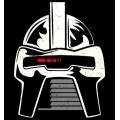ptp
Created
Updated
Index
• Overview
• Artificial Intelligence
• Audio
• Camera
• Digital
• Games
• General
• Gps
• Graphs
• Hbridge
• I2c
• Infrared Distance
• Misc
• Mobile
• Pwm
• Rafiki
• Scripting
• Servo
• Third Party Robots
• Touch Tablet
• Ultrasonic Distance
• Virtual Reality

## Depthsensor4. The installer will add this control to EZ-Builder.

Addional Setup Notes for Asus Xtion:
Please confirm the following windows environment variables:

#### `Code:`

``OPENNI2_REDIST=C:\Program Files (x86)\OpenNI2\Redist\NITE2_REDIST=C:\Program Files (x86)\PrimeSense\NiTE2\Redist\PATH=...;C:\Program Files (x86)\PrimeSense\NiTE2\Redist\;C:\Program Files (x86)\OpenNI2\Redist\;``

Create a NITE.INI file with the following content:
`Code:[General]DataDir=C:\Program Files (x86)\PrimeSense\NiTE2\Redist\NiTE2[Log]; 0 - Verbose; 1 - Info; 2 - Warning; 3 - Error. Default - NoneVerbosity=0LogToConsole=1LogToFile=1`
save in your EZ-Builder location e.g. C:\Program Files (x86)\EZ-Robot Inc\EZ-Builder\

Plugin Main Area:Left:
The picture panel where is displayed the skeleton tracking.
Right:
Run-time variables (checked for preview) values
Top:
Configuration button.
Selected Device (Configuration Form)
Connect Checkbox (dis)connects the selected device
Servos Attached Checkbox (dis)connects the servos (previous configured)

Configuration Form: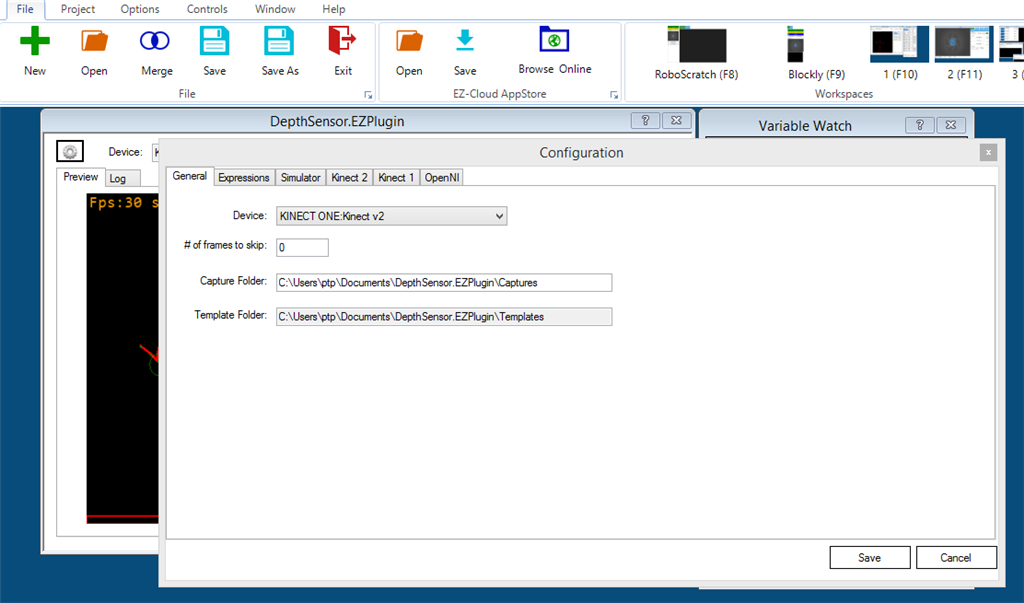*** Do not forget to press Save Button ***

Expressions: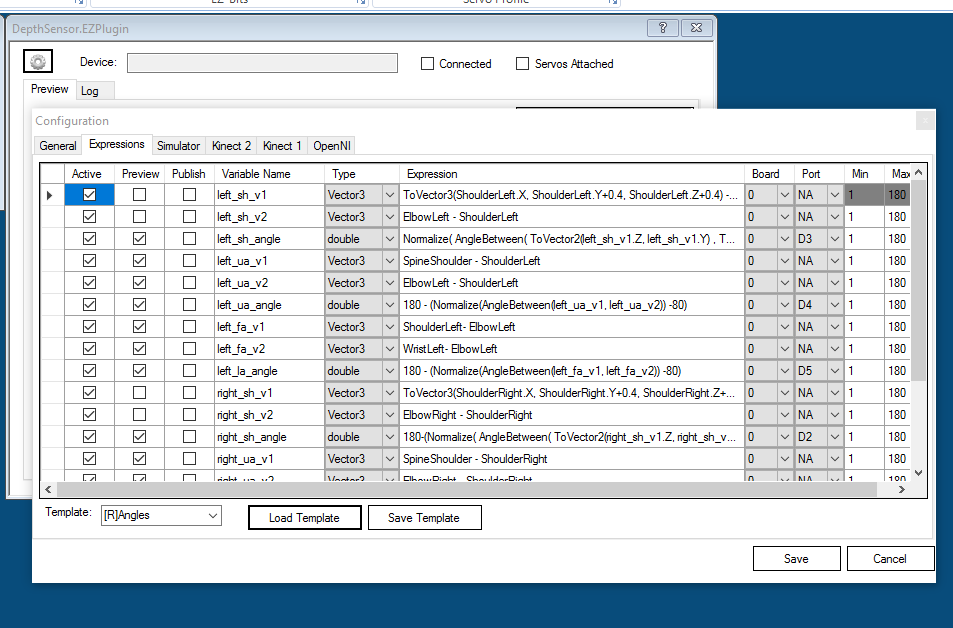Active - Enable or disables the expression
Preview - if checked the value is displayed in the main form
Publish - the variable is created and updated and can be used with EZ-Scripts
Type - There are 4 types of variables: double, Vector2 (X,Y), Vector3(X,Y,Z) and Quaternions (X,Y,Z,W)
Expression - C# expression to be compiled, evaluated and assigned to a variable.
Board, Port, Min, Max - When all specified a servo Position command will be executed using the value (double) from the expression.

Grid lines:
Drag and drop is allowed
Multiple line selection is allowed
Right Button presents a context menu option to delete expressions.

Template:
There is a default template [R]Angles, press Load template to populate the JD's upper body expressions.
Specifying a name allows to save a Template (Expressions plus last body values).

For each Joint there are two variables one for Position and another for the Rotation.

Each device returns a specific number of joints (check the device tabs), if not supported the Joint variables will be zero.

Position is a Vector3(x,y,z) representing the distance from the sensor point of view. The unit values are in meters.

Rotations is a Quaternion(x,y,z,w) is possible to obtain the Euler Angles (roll,pitch,yaw). I'm still working to unify the results, some sensors the rotations are absolute, others are relative.

For each Joint two variables are defined - For SpineBase:
SpineBase (Vector3)
QSpineBase (Quaternion)

if you want to use the SpineBase depth distance you use SpineBase.Z

Available Functions:
Vector2 ToVector2(double x, double y)
Vector3 ToVector3(double x, double y, double z)
Vector3 GetEuler(Quaternion q)
double AngleBetween(Vector2 v1, Vector2 v2)
double AngleBetween(Vector3 v1, Vector3 v2)
double Normalize(double value, double start = 0, double end = 360)
double Abs(double value)
double Min(double a, double b)
double Max(double a, double b)
double Pow(double a, double b)
double Sqrt(double d)
double Cos(double a)
double Sin(double a)
double Tan(double d)
double Cosh(double a)
double Sinh(double a)
double Tanh(double d)
double Acos(double d)
double Asin(double a)
double Atan(double a)
double Atan2(double y, double x)

*** Do not forget to press Save Button ***

Simulator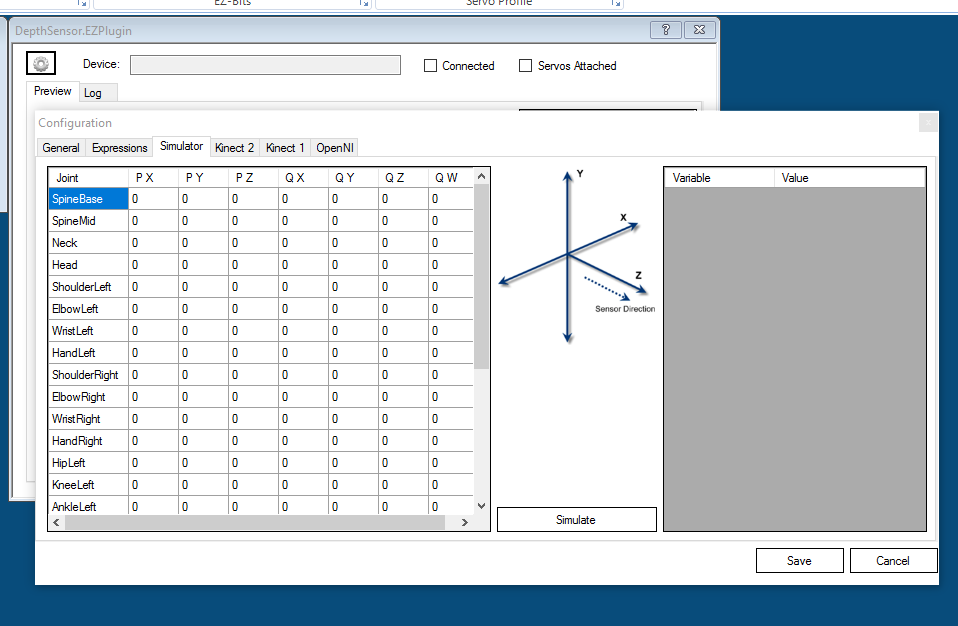Calculations are evaluated based on the Grid input:
Joint's Position values (Px,Py,Pz)
Quaternion values (Qx,Qy,Qz,Qw) only required if the used in the expressions.